# Learning Electronics

Learn to build electronic circuits

# Batteries

### Question 1:

If I wanted to build a battery-powered electric drill, would it be better to use primary cell batteries, or secondary cell batteries? Explain your answer.
"Secondary" cell batteries would be better to use for an application such as an electric drill, because it would be wasteful to have to discard so many primary-cell batteries at the rate they would be "consumed" powering such a device.

Notes:
Basically, the point of this question is to get students to research what the difference is between a primary and a secondary cell. Framing the question in a practical context makes it more interesting, and involves other practical issues as well (economics, conservation, etc.).

### Question 2:

Identify some common "primary" cell types, as well as some common ßecondary" cell types. For each of these battery types, identify their standard output voltages.
Primary cell types and voltages:
Carbon-zinc: 1.5 volts
Alkaline: 1.5 volts
Lithium: 1.8 to 3.65 volts, depending on exact chemical makeup
Mercuric-oxide: 1.35 to 1.4 volts
Secondary cell types and voltages:
Lead-acid: 2.1 volts
Nickel-cadmium: 1.2 volts
Silver-zinc: 1.5 volts
Follow-up question: what are some of the safety and environmental concerns regarding the chemical substances used in batteries, both primary and secondary types?

Notes:
Challenge your students to list a few cell types other than what is listed in the answer section.
Note: data for typical cell output voltages taken from chapter 20 of the Electronic Engineer's Reference Book, 5th edition (edited by F. Mazda).

### Question 3:

Batteries store energy in the form of chemical bonds between atoms. When a complete circuit is connected to the terminals of a battery, a current begins inside the battery and through the circuit. Chemical reactions begin inside the battery at the same time the circuit is formed, fueling this current. When all the chemicals have been depleted, though, the current will cease. This is what happens when a battery "dies."
Explain what takes place when a battery is recharged, and what electrical device(s) must be connected to a battery to recharge it. Be as specific as possible in your answer.
Recharging is a reversal of the chemical reactions taking place during the discharge of a battery. In order for this to occur, some external source of energy must force current backward through the battery.

Notes:
It is important to note to your students that not all battery types lend themselves to recharging, even though many "primary" cells can be rejuvenated a small degree by being put through a charging (reverse-current) cycle.

### Question 4:

Draw a schematic diagram showing a DC generator charging a battery, and another schematic showing a battery discharging into a resistive load. Be sure to include arrows in your drawing showing the direction of charging current.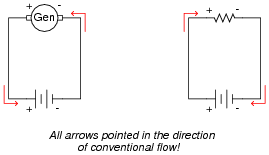Follow-up question #1: how must the battery and generator voltages compare in order for the battery to have current forced "backward" through it?
Follow-up question #2: which is the source component, and which is the load component in a circuit where a generator charges a secondary-cell battery? Explain your reasoning.

Notes:
It is very important for students to understand the direction of current through a battery, both when charging and when discharging. A helpful perspective on this topic is to think of the battery either as being a source of electrical energy versus being a load. Under which conditions (charging or discharging) is the battery playing the role of source versus load?

### Question 5:

What does the amp-hour capacity of a chemical battery represent? What does it mean if a battery is rated at, say, 150 amp-hours?
The amp-hour rating of a battery is an approximate indication of it's useful lifespan per charge.
Follow-up question: why not just rate batteries in terms of hours, instead of amp-hours? Since time is the value we are most likely interested in, why bother to refer to current (amps) at all?

Notes:
Although the basic concept of an amp-hour battery rating is simple, it is important to realize that real battery discharge characteristics are very nonlinear, and therefore the simple ämps times hours" approach may yield grossly inaccurate predictions regarding battery life.

### Question 6:

What is the best-performing secondary cell technology today, in terms of energy stored per unit mass?
There is no point in giving an answer here, as it will change as battery technology evolves. However, in your answer you need to specify an actual numerical figure for energy density, for comparison against other classmates' research!

Notes:
Questions like this are fun, because they allow the students to do research on state-of-the-art technology. Ask them where they obtained their information for the answer!

### Question 7:

Suppose I desired to power the light bulbs in a remote cabin with solar power, charging a large battery bank. The battery bank needs to be able to power ten 60-watt light bulbs for eight hours without being recharged. The system voltage is 115 volts. How large must the battery bank be, in total amp-hours?
At least 41.7 amp-hours of capacity is needed, at 115 volts.

Notes:
Ask your students what they would recommend for a battery bank capacity if they were designing this system. Would they specify 41.7 amp-hours exactly? Why or why not?

### Question 8:

Suppose a 12 volt lead-acid battery has an internal resistance of 20 milli-ohms (20 mW):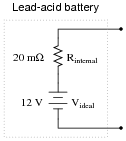If a short-circuit were placed across the terminals of this large battery, the fault current would be quite large: 600 amps!
Now suppose three of these batteries were connected directly in parallel with one another: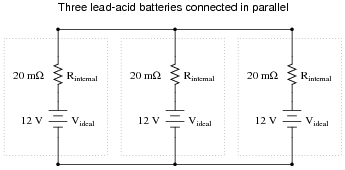Reduce this network of parallel-connected batteries into either a Thévenin or a Norton equivalent circuit, and then re-calculate the fault current available at the terminals of the three-battery "bank" in the event of a direct short-circuit.
Ifault = 1800 amps
Follow-up question: explain what practical importance this question has for parallel-connected batteries, and how either Thévenin's or Norton's theorems makes the concept easier to explain to someone else. What safety issues might be raised by the parallel connection of large batteries such as these?

Notes:
Ask your students whether they used Thévenin's Theorem or Norton's theorem to solve for the fault current. Have students demonstrate the analysis both ways to see which is easiest to understand.

### Question 9:

This heavy-duty battery test circuit seems to have a problem. Instead of the voltmeter drooping when the test switch is closed, it remains steady regardless of switch state. This happens even when the battery under test is known to be weak: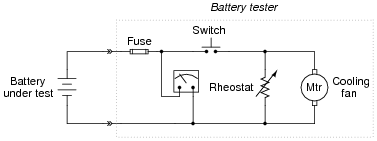Other clues to the problem include the fact that rheostat setting seems to make no difference in the voltmeter's indication, and also that the cooling fan motor does not come on when the switch is pressed.
Identify possible faults in this circuit that could account for the symptoms listed here. Also, explain why each of your proposed faults would cause the observed symptoms.
Possible faults include broken wires or open connections at the following points in the circuit (marked with "X"):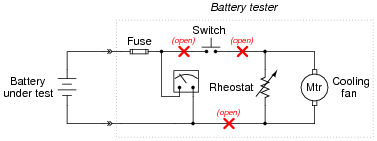Note: there is one more fault possibility that I have not listed here!

Notes:
Discuss this simple circuit with your students, asking them how they diagnosed the problem and what possible faults could account for it. Encourage them to explore more than one possibility in their diagnoses!
In case a student happens to ask what the cooling fan is there for, tell them that it is there to provide cooling to the rheostat when in use.

### Question 10:

Some brands of dry-cell batteries come equipped with a built-in charge indicator in the form of a thermal strip along one side. Pressing two white dots closes a circuit, which heats up the strip and indicates battery charge by changing color.
Compare the accuracy of a charge indicator of this general design against using a voltmeter to measure open-circuit battery voltage. Which method of measurement is a more accurate indication of battery charge, and why?
The thermal strip charge indicator is actually a more accurate indication of battery charge than an open-circuit voltage test.
Challenge question: in the absence of such a "charge indicator" on the side of a battery, how could you perform an accurate assessment of battery charge?

Notes:
This question is practical because I've seen students measure the open-circuit voltage of a battery and declare it "good" when it is really past the end of its useful life. This is not to say that a plain voltmeter is useless for determining battery charge. Obviously if an open-circuit voltage test yields abnormally low voltage, we know the battery is dead. The question is, under what condition(s) is a "good" voltage measurement a reliable indicator of adequate battery charge?

### Question 11:

What does it mean if a battery, or a bank of batteries, receives a floating charge? What does it mean if a battery, or a bank of batteries, receives an equalizing charge?
A "floating" charge is a very low charge rate, just enough to maintain a state of full charge despite normal load fluctuations on the power bus. An ëqualizing" charge is a state of overcharging, done to equalize the chemistry in all cells of a battery.
Follow-up question: qualitatively speaking, at what voltage should the power supply be set to in order to "float" a battery bank, versus in order to ëqualize" a battery bank?

Notes:
Being able to identify these types of charge strategies is important, because many battery-powered systems with automatic chargers are either designed to charge in a particular way, or have the ability to switch between different charging modes. Failure to properly charge a battery, or a battery bank, will lead to decreased performance and/or decreased life.

### Question 12:

An important equation in predicting the capacity of a lead-acid cell is called Peukert's formula:

 In t = C

Where,
I = Discharge current, in amps
n = Constant, particular to the cell (typically between 1.1 and 1.8)
t = Time of discharge
C = Constant, particular to the battery (varies with the amp-hour capacity)
Explain how Peukert's formula relates to the simpler ämp-hour" formula.
Also, calculate the discharge time for a battery with an n value of 1.2 and a C value of 200, given a discharge current of 25 amps.
The ämp-hour" formula is linear, while Peukert's formula is not (at least for values of n not equal to unity).
The discharge time for the example problem is 4.2 hours.

Notes:
Ask one of your students to write Peukert's equation on the whiteboard in front of class, along with the standard amp-hour formula. Viewed side-by-side, there is but one difference between the two equations, and it is the exponent n.

### Question 13:

A battery has an advertised capacity of 600 amp-hours, taken from tests performed over an 8-hour period (where capacity in amp-hours = It). According to Peukert's formula, what rate of current discharge will result in the battery fully discharging in 3 hours (assume an n value of 1.35)?
155 amps

Notes:
Ask your students to calculate the amount of current predicted by the ßimple" amp-hour equation for a discharge time of 3 hours. In your students' estimation, which is a more realistic prediction, Peukert's formula or the amp-hour (It) formula?

### Question 14:

An oft-repeated claim is that lead-acid batteries self-discharge at a greater rate while sitting on a concrete floor, than when installed in an automobile, or on a shelf, or in some other place where there is no direct contact with a concrete surface. Is this claim true, or not?
Design an experiment to test the veracity of this claim, and design it in such a way that a single instance of a defective battery does not skew the results of the test.
This is a fun topic to discuss! Do your research, and come to discussion time prepared with ideas on how to test this claim.

Notes:
I have heard this claim many, many times, but I have not yet been in such a position where I could test it myself. Even if I had tested it, I still wouldn't give away the answer! There are many practical matters that must be addressed in the setup and testing of batteries for self-discharge. This question provides an excellent opportunity to discuss scientific method with your students, including such principles as double-blind testing, test and control groups, and statistical correlation.